You are a hunter in a forest. In an unknown direction to you but in known distance 1 is passes a street which is a straight line. What is the smartest way to walk in order to definitely reach the street? (Photo above: 360 degree panorama on Cape Ann, Winter 2008/2009)

## Curve optimization problems

Oliver Knill, March 10-25, 2009
 Problem with shortest curve Remark Best 2D Best 3D Known to be optimal in 2D starts at 0, convex hull contains D Hunter in forest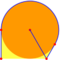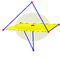No of width 1 Sailor in fog. Asteroid survey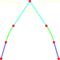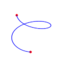Yes  with convex hull containing D Convex hull problem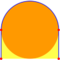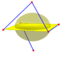Yes  closed with convex hull D Unique solution is the circle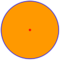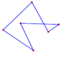Yes closed of width 1 Curves of equal width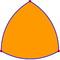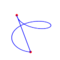Yes. Not unique
Andrew Ostergaard shared with me on March 6 a variational problem which he thinks has first appeared as an interview problem. The problem can be traced to  (1980).

What is the shortest curve in the plane starting at the origin, which has a convex hull containing the unit disc?

The problem can be embedded into a little story: you find yourself blindfolded in a forest, in distance 1 to a street. This straight line and located in an unknown direction to you. How do you walk best to reach the street for sure?

For all solution attempts, I see that the convex hull of the curve consists of pieces of the perimeter or straight lines and there are only finitely many points, where the curve is discontinuous.

The best solution, I have found so far is 6.39724 by looking at a two parameter family F(a,b) of curves, where -a is the x coordinate of the left leg and the b is x coordinate of the second leg. In order to have a minimum, grad(F) has to be zero. This solution is shown below.

 Extremizing the problem on this two dimensional plane of curves is a multivariable calculus problem: extremize the function F: ```F[{a_,b_}]:=a+2Pi-2ArcTan[a]+b-2ArcTan[b]+Sqrt[1+b^2] {a0,b0} = {a,b} /. Solve[ {D[F[{a,b}],a]==0,D[F[{a,b}],b]==0},{a,b}][]; F[{a0,b0}] ``` Gives a=1, b = 1/sqrt(3) as a critical point, so that the minimum is 1+Sqrt + 7 Pi/6 = 6.39724 ..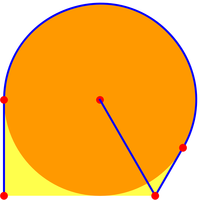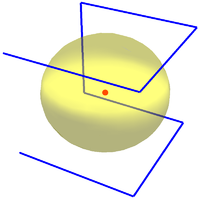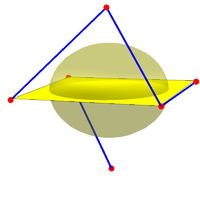The problem has obvious generalizations to other dimensions or other convex sets: find the shortest curve in space whose convex hull includes the unit ball. One obvious guess is to go along a cube and get a curve of length 14 which has as a convex hull the cube of side length 2. Added March 17: a shorter solution draws along an octahedron of side length 2 sqrt(3)/sqrt(2) enclosing the unit ball. Its length is 10sqrt(3)/sqrt(2)=12.247... If we insist on starting at the origin the length is 10sqrt(3)/sqrt(2)+sqrt(2)=13.6616... Thats the best solution I know about the 3D wall street problem: you are in space and a plane is located in distance 1 to you but in an unknown direction. How do you have to fly best to reach the plane for sure?

The unit disc or unit ball can be replaced by any other convex set like the unit square, triangle, or a polyhedron in space.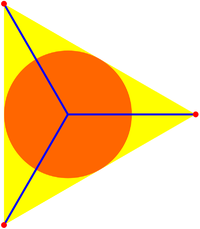A different problem is to find the minimal tree which has as a convex hull the unit disc. Here one can improve 4 sqrt(2) (the union of the two large diagonals) by connecting the center to the edges of a equilateral triangle, a tree of total length 6 (see picture to the left). This looks like a configuration which is hard to beat due to its symmetry. But it can be reduced to 2+pi (see picture to the right), which is also the best known solution to the convex enclosure problem (it is known to be the best ).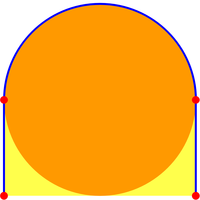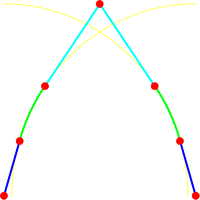The family of yourts described in this article. Move the mouse over the picture to see a deformation [Mov] Finally, there is a relation to the River Shore problem or Sailor in the fog puzzle by Ogilvy in 1972 , which asks find the shortest curve which has width 2. You are in a river of width 2 and want to reach one of the shores. What is the best way to go? This is not the same problem. The convex hull of this curve does not contain the unit disc. There are many regions of width 2 which do not contain the unit disc. The river shore problem is to find the path which will guarantee to reach one of the two shores of the river. The three dimensional version of the river shore problem is called the asteroid surveying problem . Also here, one can have a shorter curve. .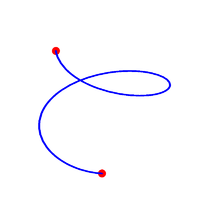An attempt to find the shortest path for the asteroid surveying problem as described in this article. [Mov]

Back to the original problem. Here is some heuristics to find the so far best solution above. (It is interesting also to investigate and monitor the search itself):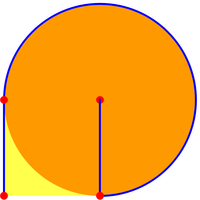Go to the boundary of the disc, then loop by 3pi/2, then go straight for a distance of 1. The minimal distance is 2+3pi/2 = 6.71239...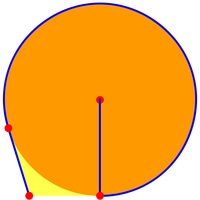This can not be improved by adjusting the leg because f(a) = a+1+2pi - 2 arctan(a) has a minimum for a=1.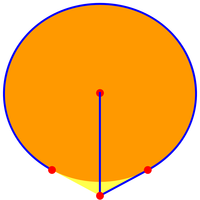Move to a point A in distance sqrt(1+a^2) away from where you are, turn around on the boundary of the disc until you see the point again. 2pi - 2 arctan(a) + a + sqrt(1+a^2) .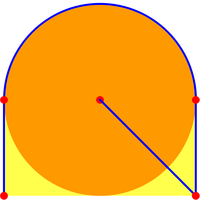Go straight away for a distance of sqrt(2), then distance 1 tangential to the boundary of the disc, loop by pi then again straight for a distance of 1. The minimal distance is 2+sqrt(2) + pi =6.55581...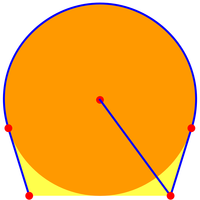Path to (a,-1), then tangential, a long circle to (-c,d) then to (-a,0). Minimizing f(a) = sqrt(1+a^2) + 2 a + 2pi - 4 arctan(a) gives a=sqrt( (9-sqrt(33))/6 ) =0.7359 ... which leads to f(a) = 6.45891...The solution above can be a bit improved to 6.39724 ... = 1+sqrt(3) + 7 pi/6 by minimzing sqrt(1+a^2)+1+a+3Pi/2-2 arctan(a). It is a mixture of the last two solutions.

The problem is related to the following problem:

Find the shortest curve in the plane such that its convex hull contains the unit disc.

 If C is a convex set, we can define r(C) = minG L(G)/L(C), where L(G) is the length of a curve whose convex hull contains C and L(C) is the length of the boundary of C? Examples: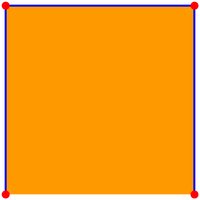• r(disc) ≤ 1/2 + 1/Pi = 0.818...
• r(x2/a2+y2/b2-1=0) ≤ 1/2 + 2(min(a,b)/L(C)
• r(square) ≤ 3/4 = 0.75
• r(equilateral triangle) ≤ 2/3 = 0.666...
• r(regular n-gon) ≤ 1-1/n and ≤ 1/2 + 1/Pi

Is the disc the convex set which maximizes r(C)?

A theoretical variational approach is probably difficult: for the original problem: we can set up some function space X of all curves r(t) which start at the origin contains a closed set X of all curves whose convex hull contains the unit disc. The functional L(c), which assigns to a curve its arc length is continuous on X. It has an infimum on X. Mathematicians would certainly like to know whether it does have a minimum in the class of all continuous functions and if yes, whether the minimium is unique modulo obvious symmetries. The problem to minimize the length of a closed curve of equal width shows that such problems could have infinite dimensional sets of minima.
• amateur math - professional math: while this is a archetype of an "amateur mathematics problem", such problems can open the door to new mathematics. I could imagine that this convex optimization problem could lead to a new branch of calculus of variations, where the traditional methods of Euler,Jacobi etc are rather helpless.
• aquiry-inquiry dilemma: when working on a mathematical problem, even if it is a toy problem like this, there is the dilemma, how to balance inquiry and aquiry. Inquiry means to work on the problem and deliberately blocking out any sources, especially not do any library - or heaven forbid, a web search and certainly not ask other mathematicians for help. Such an approach most likely would lead to elegant solutions or proofs. As in illustration, I tell about my own approach to the quest to find the shortest curve which has the unit disc in the convex hull: the curve starts at A and ends at B and line connecting these two points is tangent to the disc. The best solution of a curve from A to B which encloses D is obtained by pulling a rope from A to B around D and pulling it tight. We have now two parameters to vary, the distance of A to O, as well as the distance from B to O. Calculus shows the optimum is |A|=|B|=sqrt(2). This is heuristic only because it is not clear whether the shortest curve from A to B having D in the convex hull has to go around D.
An aquiry solution is to search aggressively what has been done already, ask other mathematicians and get so fast to the top of the knowledge. But reading or learning a solution is by far less fun than to discover it. For the convex optimization problem, the proof in  gives relief in one of the problems. Both approaches are important. A researcher, who would like to make progress on solving higher dimensional convex optimization problems like finding the shortest curve in R4 which contains the unit ball in its convex hull, must look at the sources too. I guess that there are dozens, if not hundreds of papers around which are relevant, if not crucial to make progress on such a problem. Consulting with other mathematicians certainly would accelerate the search.
Literature:
Document history:
• March 10, 2009, Posted document
• March 17, 2009, Better solution for 3D problem and graphics for 3D problem
• March 18, 2009, Literature about related river shore problem and adding to intro
• March 21, 2009, Pictures of the Yourt and 3D spiral solution and summary box
• March 22, 2009, Found reference  and probably earliest treatment  of forest problem (1980)
• March 25, 2009, Got finally a used copy of the book . See the entry about the problem. The entry shows that the convex hull problem (one shore version) had been asked also by Ogilvy.
• March 26, 2009, Added final remark
• April 10, 2009, An interesting article in slate on "Wall street problems".
• December 18, 2010, Ping Ngai CHUNG won a silver medal at the The Hang Lung Mathematics Awards is a biennial mathematics research competition for secondary school students in Hong Kong with work on this problem.
• October 2, 2019, A translation of Joris article by Steven Finch [ArXiv]